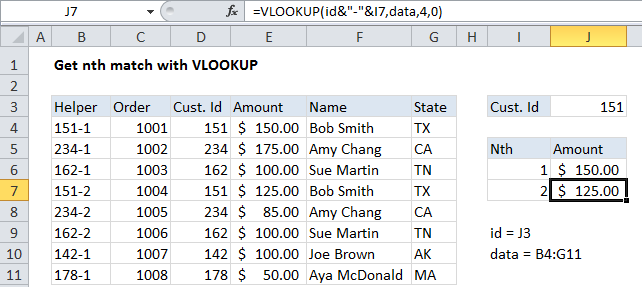## Excel Office

Excel How Tos, Tutorials, Tips & Tricks, Shortcuts

# Get nth match with VLOOKUP in Excel

This tutorial shows how to Get nth match with VLOOKUP in Excel  using the example below;

## Formula

`=VLOOKUP(id_formula,table,4,0)`Explanation

To get the nth MATCH with VLOOKUP, you’ll need to add a helper column to your table that constructs a unique id that includes the count. If this isn’t practical, you can use an array formula based on INDEX and MATCH instead.

`=VLOOKUP(id&"-"&I6,data,4,0)`

### How this formula works

This formula depends on a helper column, which is added as the first column to the source data table. The helper column contains a formula which builds a unique lookup value from an existing id and a counter. The counter counts the number of times the unique id has appeared in the data table.

Worked Example:   Create hyperlink with VLOOKUP in Excel

In the example, the formula cell B4 of the helper column looks like this:

`=D4&"-"&COUNTIF(\$D\$4:D4,D4)`

This formula picks up the value in D4 and uses concatenation to add a hyphen, and the result of a COUNTIF function. The COUNTIF function uses an expanding range (the mixed reference \$D\$4:D4) to generate a running count of the id in the data.

Worked Example:   VLOOKUP without #N/A error in Excel

On the lookup side, VLOOKUP is used to fetch values form the table, taking into account the “nth” occurrence. The trick is to build a lookup value with the same structure as the values that appear in the helper column, taking into account “nth”. Again, this is done with concatenation, by adding a hyphen and a value that represents “nth” to a normal lookup value (pulled from the named range “id” in this case).

Worked Example:   One way to track attendance using Excel formula